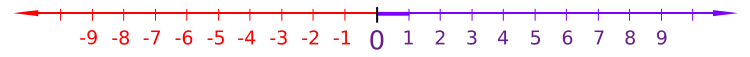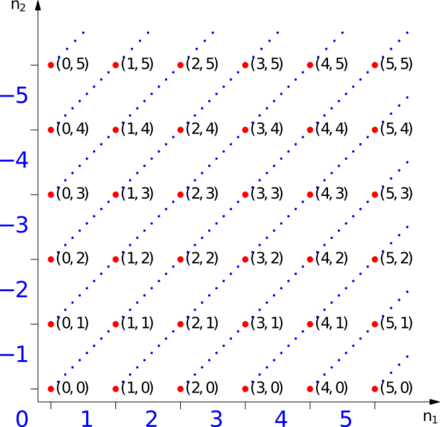# Integer

An integer (from the Latin integer meaning "whole")[note 1] is a number that can be written without a fractional component. For example, 21, 4, 0, and −2048 are integers, while 9.75, 5 12, and 2 are not.

The set of integers consists of zero (0), the natural numbers (1, 2, 3, …), also called whole numbers or counting numbers, and their additive inverses (the negative integers, i.e. −1, −2, −3, …). This is often denoted by a boldface Z ("Z") or blackboard bold(Unicode U+2124 ℤ) standing for the German word Zahlen ([ˈtsaːlən], "numbers"). ℤ is a subset of the sets of rational and real numbers and, like the natural numbers, is countably infinite.

The integers form the smallest group and the smallest ring containing the natural numbers. In algebraic number theory, the integers are sometimes called rational integers to distinguish them from the more general algebraic integers. In fact, the (rational) integers are the algebraic integers that are also rational numbers.

## Algebraic propertiesIntegers can be thought of as discrete, equally spaced points on an infinitely long number line. In the above, non-negative integers are shown in purple and negative integers in red.

Like the natural numbers, Z is closed under the operations of addition and multiplication, that is, the sum and product of any two integers is an integer. However, with the inclusion of the negative natural numbers, and, importantly, 0, Z (unlike the natural numbers) is also closed under subtraction. The integers form a unital ring which is the most basic one, in the following sense: for any unital ring, there is a unique ring homomorphism from the integers into this ring. This universal property, namely to be an initial object in the category of rings, characterizes the ring Z.

Z is not closed under division, since the quotient of two integers (e.g. 1 divided by 2), need not be an integer. Although the natural numbers are closed under exponentiation, the integers are not (since the result can be a fraction when the exponent is negative).

The following lists some of the basic properties of addition and multiplication for any integers a, b and c.

Properties of addition and multiplication on integers
Closure: a + b is an integer a × b is an integer
Associativity: a + (b + c) = (a + b) + c a × (b × c) = (a × b) × c
Commutativity: a + b = b + a a × b = b × a
Existence of an identity element: a + 0 = a a × 1 = a
Existence of inverse elements: a + (−a) = 0 The only invertible rational integers (called units) are −1 and 1.
Distributivity: a × (b + c) = (a × b) + (a × c) and (a + b) × c = (a × c) + (b × c)
No zero divisors: (*) If a × b = 0, then a = 0 or b = 0 (or both)

In the language of abstract algebra, the first five properties listed above for addition say that Z under addition is an abelian group. As a group under addition, Z is a cyclic group, since every non-zero integer can be written as a finite sum 1 + 1 + … + 1 or (−1) + (−1) + … + (−1). In fact, Z under addition is the only infinite cyclic group, in the sense that any infinite cyclic group is isomorphic to Z.

The first four properties listed above for multiplication say that Z under multiplication is a commutative monoid. However, not every integer has a multiplicative inverse; e.g. there is no integer x such that 2x = 1, because the left hand side is even, while the right hand side is odd. This means that Z under multiplication is not a group.

All the rules from the above property table, except for the last, taken together say that Z together with addition and multiplication is a commutative ring with unity. It is the prototype of all objects of such algebraic structure. Only those equalities of expressions are true in Z for all values of variables, which are true in any unital commutative ring. Note that certain non-zero integers map to zero in certain rings.

At last, the property (*) says that the commutative ring Z is an integral domain. In fact, Z provides the motivation for defining such a structure.

The lack of multiplicative inverses, which is equivalent to the fact that Z is not closed under division, means that Z is not a field. The smallest field with the usual operations containing the integers is the field of rational numbers. The process of constructing the rationals from the integers can be mimicked to form the field of fractions of any integral domain. And back, starting from an algebraic number field (an extension of rational numbers), its ring of integers can be extracted, which includes Z as its subring.

Moreover, Z is a principal ring.

Although ordinary division is not defined on Z, the division "with remainder" is defined on them. It is called Euclidean division and possesses the following important property: that is, given two integers a and b with b ≠ 0, there exist unique integers q and r such that a = q × b + r and 0 ≤ r < |b|, where |b| denotes the absolute value of b. The integer q is called the quotient and r is called the remainder of the division of a by b. The Euclidean algorithm for computing greatest common divisors works by a sequence of Euclidean divisions.

Again, in the language of abstract algebra, the above says that Z is a Euclidean domain. This implies that Z is a principal ideal domain and any positive integer can be written as the products of primes in an essentially unique way. This is the fundamental theorem of arithmetic.

## Order-theoretic properties

Z is a totally ordered set without upper or lower bound. The ordering of Z is given by: :… −3 < −2 < −1 < 0 < 1 < 2 < 3 < … An integer is positive if it is greater than zero and negative if it is less than zero. Zero is defined as neither negative nor positive.

The ordering of integers is compatible with the algebraic operations in the following way:

1. if a < b and c < d, then a + c < b + d
2. if a < b and 0 < c, then ac < bc.

It follows that Z together with the above ordering is an ordered ring.

The integers are the only nontrivial totally ordered abelian group whose positive elements are well-ordered. This is equivalent to the statement that any Noetherian valuation ring is either a field or a discrete valuation ring.

## ConstructionRed points represent ordered pairs of natural numbers. Linked red points are equivalence classes representing the blue integers at the end of the line.

In elementary school teaching, integers are often intuitively defined as the (positive) natural numbers, zero, and the negations of the natural numbers. However, this style of definition leads to many different cases (each arithmetic operation needs to be defined on each combination of types of integer) and makes it tedious to prove that these operations obey the laws of arithmetic. Therefore, in modern set-theoretic mathematics a more abstract construction, which allows one to define the arithmetical operations without any case distinction, is often used instead. The integers can thus be formally constructed as the equivalence classes of ordered pairs of natural numbers (a,b).

The intuition is that (a,b) stands for the result of subtracting b from a. To confirm our expectation that 1 − 2 and 4 − 5 denote the same number, we define an equivalence relation ~ on these pairs with the following rule:precisely whenAddition and multiplication of integers can be defined in terms of the equivalent operations on the natural numbers; denoting by [(a,b)] the equivalence class having (a,b) as a member, one has:The negation (or additive inverse) of an integer is obtained by reversing the order of the pair:Hence subtraction can be defined as the addition of the additive inverse:The standard ordering on the integers is given by:iffIt is easily verified that these definitions are independent of the choice of representatives of the equivalence classes.

Every equivalence class has a unique member that is of the form (n,0) or (0,n) (or both at once). The natural number n is identified with the class [(n,0)] (in other words the natural numbers are embedded into the integers by map sending n to [(n,0)]), and the class [(0,n)] is denoted n (this covers all remaining classes, and gives the class [(0,0)] a second time since −0 = 0.

Thus, [(a,b)] is denoted byIf the natural numbers are identified with the corresponding integers (using the embedding mentioned above), this convention creates no ambiguity.

This notation recovers the familiar representation of the integers as {…, −2, −1, 0, 1, 2, …}.

Some examples are:## Computer science

An integer is often a primitive data type in computer languages. However, integer data types can only represent a subset of all integers, since practical computers are of finite capacity. Also, in the common two's complement representation, the inherent definition of sign distinguishes between "negative" and "non-negative" rather than "negative, positive, and 0". (It is, however, certainly possible for a computer to determine whether an integer value is truly positive.) Fixed length integer approximation data types (or subsets) are denoted int or Integer in several programming languages (such as Algol68, C, Java, Delphi, etc.).

Variable-length representations of integers, such as bignums, can store any integer that fits in the computer's memory. Other integer data types are implemented with a fixed size, usually a number of bits which is a power of 2 (4, 8, 16, etc.) or a memorable number of decimal digits (e.g., 9 or 10).

## Cardinality

The cardinality of the set of integers is equal to 0 (aleph-null). This is readily demonstrated by the construction of a bijection, that is, a function that is injective and surjective from Z to N. If N = {0, 1, 2, …} then consider the function:{… (−4,8) (−3,6) (−2,4) (−1,2) (0,0) (1,1) (2,3) (3,5) …}

If N = {1, 2, 3, ...} then consider the function:{… (−4,8) (−3,6) (−2,4) (−1,2) (0,1) (1,3) (2,5) (3,7) …}

If the domain is restricted to Z then each and every member of Z has one and only one corresponding member of N and by the definition of cardinal equality the two sets have equal cardinality.

## Notes

1. Integer's first, literal meaning in Latin is "untouched", from in ("not") plus tangere ("to touch"). "Entire" derives from the same origin via French (see: Evans, Nick (1995). "A-Quantifiers and Scope". In Bach, Emmon W. Quantification in Natural Languages. Dordrecht, The Netherlands; Boston, MA: Kluwer Academic Publishers. p. 262. ISBN 0-7923-3352-7.)

## References

1. Miller, Jeff (2010-08-29). "Earliest Uses of Symbols of Number Theory". Retrieved 2010-09-20.
2. Peter Jephson Cameron (1998). Introduction to Algebra. Oxford University Press. p. 4. ISBN 978-0-19-850195-4.
3. Serge, Lang (1993), Algebra (3rd ed.), Addison-Wesley, pp. 86–87, ISBN 0-201-55540-9
4. Warner, Seth (2012), Modern Algebra, Dover Books on Mathematics, Courier Corporation, Theorem 20.14, p. 185, ISBN 9780486137094.
5. Mendelson, Elliott (2008), Number Systems and the Foundations of Analysis, Dover Books on Mathematics, Courier Dover Publications, p. 86, ISBN 9780486457925.
6. Ivorra Castillo: Álgebra
7. Frobisher, Len (1999), Learning to Teach Number: A Handbook for Students and Teachers in the Primary School, The Stanley Thornes Teaching Primary Maths Series, Nelson Thornes, p. 126, ISBN 9780748735150.
8. Campbell, Howard E. (1970). The structure of arithmetic. Appleton-Century-Crofts. p. 83. ISBN 0-390-16895-5.

## Sources

• Bell, E.T., Men of Mathematics. New York: Simon & Schuster, 1986. (Hardcover; ISBN 0-671-46400-0)/(Paperback; ISBN 0-671-62818-6)
• Herstein, I.N., Topics in Algebra, Wiley; 2 edition (June 20, 1975), ISBN 0-471-01090-1.
• Mac Lane, Saunders, and Garrett Birkhoff; Algebra, American Mathematical Society; 3rd edition (April 1999). ISBN 0-8218-1646-2.Look up integer in Wiktionary, the free dictionary.